# Chapter 4: Measurement of Angles – Exercise 4.1

## Measurement of Angles – Exercise 4.1 – Q.1

(i)   9π/5

We have,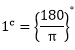Now,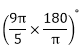= 324°

(ii)   (-5π)/6

We have,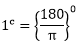Now,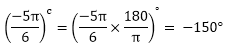(iii)  (18π/5)c

We have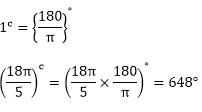Now,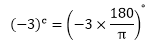(iv) We have,Now,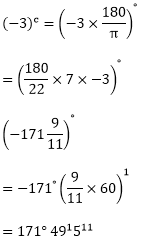(v) We have,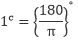Now,= 630°

(vi) We have,Now,## Measurement of Angles – Exercise 4.1 – Q.2

(i) 300°

We have, 180° = πcNow,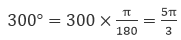(ii) 35°

We have,

180° = πc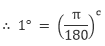Now,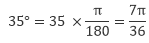(iii) – 56°

We have

180° = πc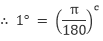Now,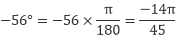(iv) 135°

We have,

180° = πcNow,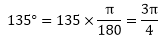(v)  – 300°

We have

180° = πc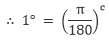Now,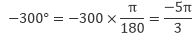(vi) 7°301

We have,

180° = πc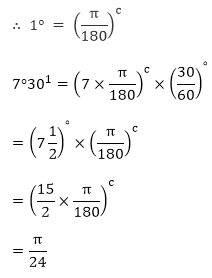(vii) 125°301

We  have

180° = πc(viii)  – 47°301

we have,

180° = πc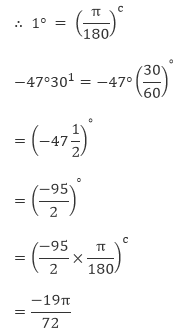## Measurement of Angles – Exercise 4.1 – Q.3

Let θ1 and θ2 be two acute angles of a right angled triangle.

∴ difference of acute angles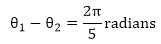∵  in a right angled triangle,On solving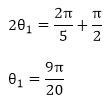From equation (ii)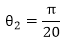So angles in degrees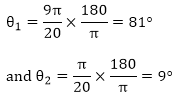## Measurement of Angles – Exercise 4.1 – Q.4

Let θ1 and θ2 and θ3 be the angle or triangle.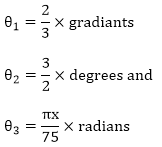Now,

We have to express all the angles in degrees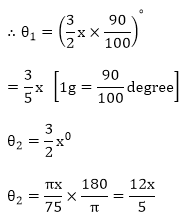By angle slam property,⟹ x = 40°

∴  θ1 = 24°, θ2 = 60°, θ3 = 96°### Course Features

• Video Lectures
• Revision Notes
• Previous Year Papers
• Mind Map
• Study Planner
• NCERT Solutions
• Discussion Forum
• Test paper with Video Solution